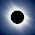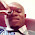# Java program to find square root of number - Example Code

Java program for square root or a number in Java
How to write Java program to find square root of a number is common Java programming exercise which many institute use in there Java course along with Java program to print Fibonacci series and How  to find Armstrong numbers in Java, which we have seen earlier.  Java program for square root is also a popular question during college semester exams and  various programming tests. If you are familiar with Java API then writing a Java program which can find square root of number using java.lang.Math class is not difficult. You just need to pass a double value and it returns a double which is square root of number you passed.  In next section we will see complete code example of finding square root of a number from Java program. Another Java coding questions which is very popular and related to programming exercise is writing Java program to find prime numbers, if you are going to appear in Java interviews then its worth looking.

## How to find Square root of a number in Java

As I said its easy to calculate square root using java.lang.Math sqrt() function but things can be tricky question, if interviewer will ask you to write your own sqrt() function during programming interviews, well its not that easy to write sqrt() function which can operatre on double but one trick I use to remember the concept that square of square root must be either less than or equal to number, you can use that concept to write your own sqrt() method in any programming language including Java. barring Java interviews, I suggest programmer to use standard JDK library or open source library like Spring, Apache etc for such common task because of quality of code and amount of testing done of those libraries. Anyway here we will see Java program for finding square root using API method,

package test;

import java.util.Scanner;

/**
*
* Java program to find square root of number in Java.
* This Java program example demonstrate using Math class
* sqrt() method to get square root of a number in Java.
*
* @author java67
*/

public class SquareRoot{

public static void main(String args[]) {

//Used to get input number for which square root to find
Scanner scanner =
new Scanner(System.in);

System.
out.println("Enter number to find square root in Java : ");

//getting input number from user to calculate square root

double square = scanner.nextDouble();

//getting square root of a number in Java

double squareRoot = Math.sqrt(square);

//printing number and its square root in Java
System.
out.printf("Square root of number: %f is : %f %n" , square, squareRoot);

}

}

Output:
Enter number to find square root in Java :
64
Square root of number:
64.000000 is : 8.000000

This was our Java program to find square root of a number in Java. It can find square root of any floating point number and you don’t need to only supply integers numbers. As I said use Java standard library methods to calculate square root but prepare with your own version of square root function if going for any programming interview.

Further Learning
Data Structures and Algorithms: Deep Dive Using Java
Java Fundamentals: The Java Language
Complete Java Masterclass

Other Java programming and homework exercise:

#### 8 comments:

1.In mathematics, a square root of a number a is a number y such that y2 = a, or, in other words, a number y whose square (the result of multiplying the number by itself, or y × y) is a.

2.Write a Java program to find Square root of a number is common programming exercise for beginners in Java. Some people use it as practice and homework exercise to learn Java programming and
get familiar with Java API in particular java.lang.Math, which holds key method for various mathematical tasks.

3.4.LET SOMEONE CORRECT THIS FOR ME. THANKS

//java program for dept...........
//group 9
//serial no 44
//name1:
//name2:
//name3:
//name4
//name5
import javax.swing.JOptionPane;
public class CSCGP9 {
public static void main (String [] args){
float z, zl, c=0.0f ;
system.out.printLn ("S/N \t number \tsquare \tcube")n;
for(int k = 1; k<= 9; k++){
c=Float.parseFloat (JOptionpane.showInputDialog("Enter a number",0));
z=square(c);
zl=cube (c);
system.out.println(k+"\t"+c+"\t"+square(c)+"\t"+cube(c));
}
}
static Float square (float x){
return x*x;
}
static Float cube (float x){
return x*x*x;
}
}

5.C:\Users\student\Desktop\19>javac Sqr2.java
Sqr2.java:10: error: possible loss of precision
float r=Math.sqrt(square);
^
required: float
found: double
1 error how to overcome this???

1.Hello @Annonymous, Math.sqrt() returns double, which is bigger data type than float hence you cannot store a double value to float variable. if you want you can use the cast operator e.g.

float r= (float) Math.sqrt(square);

This will fix the problem.

6.Java program for finding nth root

7.Its too big see this is a very small one
import java.util.*;

/*Java Program to find square, square root and cube of a given number*/
public class j5
{
public static void main(String args[]){
Scanner sc=new Scanner(System.in);
int num;

System.out.print("Enter an integer number: ");
num=sc.nextInt();

System.out.println("Square of "+ num + " is: "+ Math.pow(num, 2));
System.out.println("Cube of "+ num + " is: "+ Math.pow(num, 3));
System.out.println("Square Root of "+ num + " is: "+ Math.sqrt(num));
}
}